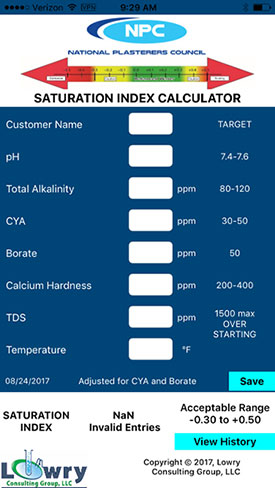# NPC LSI Calculator App

Have you downloaded the NPC LSI Calculator app? It is the most advanced and accurate calculator for determining the Langelier Saturation Index (LSI) for swimming pool and spa water. It was developed by noted pool and spa water chemistry expert and author, Robert W. Lowry. It is the only LSI calculator that currently has exact adjustments for cyanuric acid and borate.

The LSI is used to predict if pool or spa water is corrosive, balanced or scaling. The NPC LSI Calc uses the original mathematical equations which use logarithms, square roots, and interpolated constants for calculations. We do not use inaccurate lookup tables and factors. The actual water test results are used for calculations.

The NPC LSI Calc is easy to use, you input the customer or pool name and then the water test results for pH, Total Alkalinity (TA), Cyanuric Acid (CYA) Borate (B) Calcium Hardness (CH), Total Dissolved Solids (TDS), and the water Temperature (T) in degrees Fahrenheit. The LSI with adjustments is automatically calculated and displayed. The background color of the LSI value changes from green to amber to red depending on whether the water is Corrosive, Acceptable, Ideal, or Scaling. This gives a visual or at a glance notice of the water’s condition.